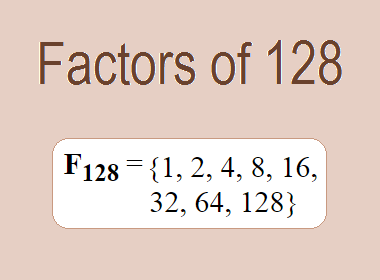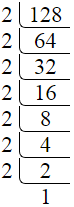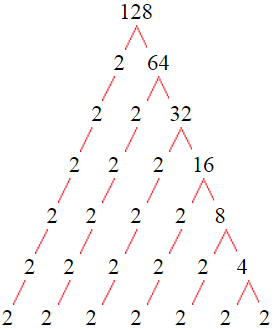# Factors of 128The factors of 128 are 1, 2, 4, 8, 16, 32, 64, and 128 i.e. F128 = {1, 2, 4, 8, 16, 32, 64, 128}. The factors of 128 are all the numbers that can divide 128 without leaving a remainder.

We can check if these numbers are factors of 128 by dividing 128 by each of them. If the result is a whole number, then the number is a factor of 128. Let's do this for each of the numbers listed above:

·        1 is a factor of 128 because 128 divided by 1 is 128.

·        2 is a factor of 128 because 128 divided by 2 is 64.

·        4 is a factor of 128 because 128 divided by 4 is 32.

·        8 is a factor of 128 because 128 divided by 8 is 16.

·        16 is a factor of 128 because 128 divided by 16 is 8.

·        32 is a factor of 128 because 128 divided by 32 is 4.

·        64 is a factor of 128 because 128 divided by 64 is 2.

·        128 is a factor of 128 because 128 divided by 128 is 1.

## How to Find Factors of 128?

1 and the number itself are the factors of every number. So, 1 and 128 are two factors of 128. To find the other factors of 128, we can start by dividing 128 by the numbers between 1 and 128. If we divide 128 by 2, we get a remainder of 0. Therefore, 2 is a factor of 128. If we divide 128 by 3, we get a remainder of 2. Therefore, 3 is not a factor of 128.

Next, we can check if 4 is a factor of 128. If we divide 128 by 4, we get a remainder of 0. Therefore, 4 is also a factor of 128. We can continue this process for all the possible factors of 128.

Through this process, we can find that the factors of 128 are 1, 2, 4, 8, 16, 32, 64, and 128. These are the only numbers that can divide 128 without leaving a remainder.

********************

********************

## Properties of the Factors of 128

The factors of 128 have some interesting properties. One of the properties is that the sum of the factors of 128 is equal to 255. We can see this by adding all the factors of 128 together:

1 + 2 + 4 + 8 + 16 + 32 + 64 + 128 = 255

Another property of the factors of 128 is that the prime factor of 128 is 2 only.

## Applications of the Factors of 128

The factors of 128 have several applications in mathematics. One of the applications is in finding the highest common factor (HCF) of two or more numbers. The HCF is the largest factor that two or more numbers have in common. For example, to find the HCF of 128 and 160, we need to find the factors of both numbers and identify the largest factor they have in common. The factors of 128 are 1, 2, 4, 8, 16, 32, 64, and 128. The factors of 160 are 1, 2, 4, 5, 8, 10, 16, 20, 32, 40, 80 and 160. The largest factor that they have in common is 32. Therefore, the HCF of 128 and 160 is 32.

Another application of the factors of 128 is in prime factorization. Prime factorization is the process of expressing a number as the product of its prime factors. The prime factor of 128 is 2 since 2 is the only prime number that can divide 128 without leaving a remainder. Therefore, we can express 128 as:

128 = 2 × 2 × 2 × 2 × 2 × 2 × 2

We can do prime factorization by division and factor tree method also. Here is the prime factorization of 128 by division method,128 = 2 × 2 × 2 × 2 × 2 × 2 × 2

Here is the prime factorization of 128 by the factor tree method,128 = 2 × 2 × 2 × 2 × 2 × 2 × 2

## Conclusion

The factors of 128 are the numbers that can divide 128 without leaving a remainder. The factors of 128 are 1, 2, 4, 8, 16, 32, 64, and 128. The factors of 128 have some interesting properties, such as having a sum of 255. The factors of 128 have several applications in mathematics, such as finding the highest common factor and prime factorization.